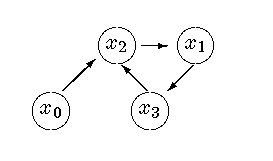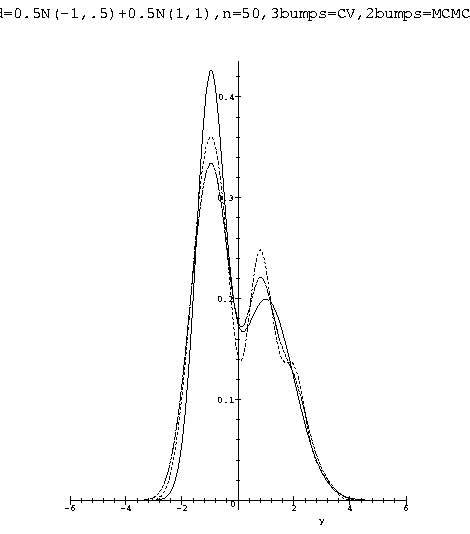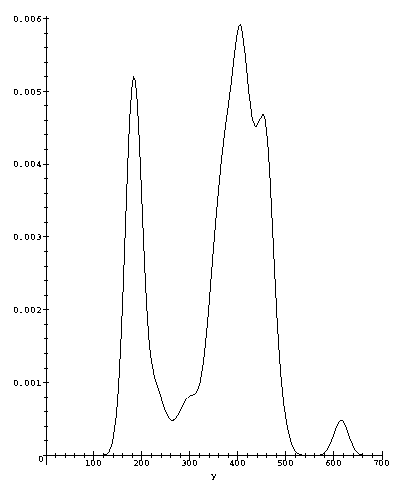# CV-NP BAYESIANISM BY MCMC

### Cross-Validated Non-Parametric Bayesianism by Markov Chain Monte Carlo## Abstract

Completely automatic and adaptive non-parametric inference is a pie in the sky. The frequentist approach, best exemplified by the kernel estimators, has excellent asymptotic characteristics but it is very sensitive to the choice of smoothness parameters. On the other hand the Bayesian approach, best exemplified by the mixture of gaussians models, is optimal given the observed data but it is very sensitive to the choice of prior. In 1984 the author proposed to use the Cross-Validated gaussian kernel as the likelihood for the smoothness scale parameter h, and obtained a closed formula for the posterior mean of h based on Jeffreys's rule as the prior. The practical operational characteristics of this bayes' rule for the smoothness parameter remained unknown for all these years due to the combinatorial complexity of the formula. It is shown in this paper that a version of the metropolis algorithm can be used to approximate the value of h producing remarkably good completely automatic and adaptive kernel estimators. A close study of the form of the cross validated likelihood suggests a modification and a new approach to Bayesian Non-parametrics in general.

## 1  Introduction

A basic problem of statistical inference is to estimate the probability distribution that generated the observed data. In order to allow the data to speak by themselves, it is desirable to solve this problem with a minimum of a priori assumptions on the class of possible solutions. For this reason, the last thirty years have been burgeoning with interest in nonparametric estimation. The main positive results are almost exclusively from non-Bayesian quarters, but due to recent advances in Monte Carlo methods and computer hardware, Bayesian nonparametric estimators are now becoming more competitive.

This paper aims to extend the idea in  to a new general Bayesian approach to nonparametric density estimation. As an illustration one of the techniques is applied to compute the estimator in . In particular it is shown how to approximate the bayes estimator (for Jeffreys' prior and quadratic loss) for the bandwidth parameter of a kernel using a version of the metropolis algorithm. The computer experiments with simulated data clearly show that the bayes estimator with Jeffreys' prior outperforms the standard estimator produced by plain Cross-validation.

## 2  Density Estimation by Summing Over Paths

Any non-Bayesian nonparametric density estimator can be turned into a Bayesian one by the following three steps:

1. Transform the estimator into a likelihood for the smoothness parameters via the sum over paths technique introduced below.

2. Put a reference prior on the smoothness parameters.

3. Use the predictive distribution as the Bayesian estimator obtainable by Markov Chain Monte Carlo.

The summing over paths method, springs from the simple idea of interchanging sums and products in the expression for the cross-validated kernel likelihood (see ). Without further reference to its humble origins, let us postulate the following sequence of functions,

 Fn = F(x0,x1,x2,Ľ,xn | h ) µ ĺ all paths n Ő j = 0 Kh(xj -xij)
(1)
where h > 0, Kh(x) = 1/hK(x/h) with K a density symmetric about 0. We call a vector of indices, (i0,Ľ,in) with the property that ij Î {0,Ľ,n}\{j}, a path (more specifically a general unrestricted path, see below). The sum above runs over all the nn+1 possible paths. The functions, Fn are defined up to a proportionality constant independent of the xj's.

Notice that by flipping the sum and the product we get

 1 nn+1 ĺ all paths n Ő j = 0 Kh(xj - xij) = f-0,n(x0) f-1,n(x1) Ľf-n,n(xn)
(2)
where,
 f-j,n(xj) = 1 n n ĺ ij ą j Kh(xj - xij).
(3)
Thus, f-j,n(xj) is nothing but the kernel density estimator of f(xj) based on all the data except the jth observation xj. Under mild regularity conditions the kernel estimator is known to converge (in every conceivable way) provided that h = hn is taken as a function of n such that, hn® 0 and n hn® Ą as n®Ą.

The Fn's can be used as a universal method for attaching a class of exchangeable one parameter models to any set of observations. The positive scalar parameter h is the only free parameter, and different models are obtained by changing the kernel function K.

These empirical parametric models are invariant under relabeling of the observations (i.e. they are exchangeable) but they do not model the observations as independent variables. Rather, these models introduce a pattern of correlations for which there is a priori no justification. This suggest that there might be improvements in performance if the sum is restricted to special subsets of the set of all nn+1 paths. Three of these modifications are mentioned in the following section.

Notice also that the ability of the Fn to adapt comes at the expense of regularity. These models are always non-regular. If the kernel has unbounded support then Fn integrates to infinity but the conditional distribution of x0 given x1,Ľ,xn and h is proper. When the kernel has compact support the Fn are proper but still non-regular since their support now depends on h.

The above recipe would have been a capricious combination of symbols if it not were for the fact that, under mild regularity conditions, these models adapt to the form of the true likelihood as n increases.

As a function of x0 = x, the Fn have the following asymptotic property,

Theorem 1 If x1,x2,Ľ,xn are iid observations from an unknown pdf f which is continuous a.s. and h = hn is taken as a function of n such that, hn® 0 and nhn® Ą as n® Ą, then,

Fn
 óő Fndx0
= f-0,n(x)+o(1) = f(x)+o(1).
(4)
where the little o is taken in probability as n® Ą

Proof (sketch only)
Just flip the sum and the product to get again,

 1 nn+1 ĺ all paths n Ő j = 0 Kh(xj - xij) = f-0,n(x) f-1,n(x1) Ľf-n,n(xn)
(5)
Under the simple regularity conditions of the theorem, the kernel estimator is known to converge in probability as n®Ą. However, even though x0 appears in all of the n+1 factors, and their number goes to infinity, still all the factors are converging to the value of the true density at the given point. Therefore the theorem follows.[¯]

It is worth noticing that the above theorem is only one of a large number of results that are readily available from the thirty years of literature on density estimation. In fact under appropriate regularity conditions the convergence can be strengthen to be pointwise a.s., uniformly a.s., or globally a.s. in L1, or L2.

## 3  Paths, Graphs and Loops

Each of the nn+1 paths (i0,Ľ,in) can be represented by a graph with nodes x0,Ľ,xn and edges from xj to xk if and only if ij = k. Here are some graphs for paths with n = 3. For example, the path (2,3,1,2) is given a probability proportional to

 Kh(x0-x2)Kh(x1-x3)Kh(x2-x1)Kh(x3-x2)
(6)
and represented by the graph in figure . Let's call it a 1-3-loop.Figure 1: The graph of (2,3,1,2)

The path (1,2,3,0) is the single ordered loop of size four (a 4-loop), (3,0,1,2) is the same loop backwards (also a 4-loop), (2,3,0,1) are two disconnected loops (a 2-2-loop) and (1,0,0,0) is connected and contains a loop of size two with x0 and x1 in it (a 1-1-2-loop). Draw the pictures!

The classification of paths in terms of number and size of loops appears naturally when trying to understand how Fn distributes probability mass among the different paths. To be able to provide simple explicit formulas let us take K in the definition of Fn to be the standard gaussian density, i.e. from now on we take,

 K(x) = (2p)-1/2exp ćç č -x2 2 ö÷ ř .
(7)
The gaussian kernel has unbounded support and that makes the total integral of each path to diverge. Thus, the partition function
 Z
 =
 óő Fndx0Ľdxn
(8)
 =
 ĺ all paths óő n Ő j = 0 Kh(xj -xij) dx0Ľdxn
(9)
is the sum of infinities and it also diverges. Recall that this anomaly is the price we need to pay for using a model with a finite number of free parameters (only one in this case) and hoping to still adapt to the form of the true likelihood as n®Ą. Even though the value of Z is in fact Ą we can still write (formally) a useful decomposition that will help explain how the Fn's adapt and how to modify the set of paths to improve the convergence. We first need the following simple property of gaussians,
 óő Ka(x-y) Kb(y-z) dy = KÖ{a2+b2}(x-z).
(10)
This can be shown by straight forward integration after completing the square. Now notice that whatever the value of the integrals appearing in equation (9) that value only depends on the type of loop that is being integrated. For this reason we omit the integrand and simply denote the value of the integral with the integral sign and the type of loop. With this notation we have,

Theorem 2

 óő m1-m2-Ľ-mk-loop = ćč óő m1-loop öř ćč óő m2-loop öř Ľ ćč óő mk-loop öř
(11)
More over, ň1-loop = 1 and for m > 1,
 óő m-loop = (2p)-1/2 h-1 Öm L
(12)
where we write formally L = ňdx.

Proof
Equation (11) follows from Fubini's theorem. To get ( 12) use Fubini's theorem and apply (10) each time to obtain,

 óő m-loop
 =
 óő Kh(x0-x1)Kh(x1 - x2)Kh(x2-x3) ĽKh(xm-1-x0) dx0dx1Ľdxm-1
 =
 óő KÖ2h(x0-x2) ĽKh(xm-1-x0)dx0dx2Ľdxm-1
 Ľ
 =
 óő K[Ö(m-1)]h(x0-xm-1)Kh(xm-1-x0) dx0dxm-1
 =
 óő KÖmh(0) dx0 = (2p)-1/2 h-1 Öm L
[¯]
Hence, by splitting the sum over all paths into,
 ĺ all paths = ĺ 2-2...-2-loops + ĺ 1-3-2...-2-loops + Ľ+ ĺ (n+1)-loops
and applying the previous theorem we obtain,
Z = N2-2Ľ-2 ć
ç
č
(2p)-1/2 h-1
Ö2
L ö
÷
ř
(n+1)/2

+ Ľ+ Nn+1 ć
ç
ç
ç
ç
č
(2p)-1/2 h-1
 ___Ön+1
L ö
÷
÷
÷
÷
ř
1

(13)
where for simplicity we have assumed that n is odd and we denote by Nm1-Ľ-mk the total number of m1-Ľ-mk-loops. Using simple combinatorial arguments it is possible to write explicit formulas for the number of loops of each kind. The important conclusion from the decomposition (13) is that even though the Fn appear to be adding equally over all paths, in reality they end up allocating almost all the probability mass on paths with maximally disconnected graphs. This is not surprising. This is the reason why there is consistency when assuming iid observations. There is a built in bias towards independence. The bias can be imposed explicitly on the Fn by restricting the paths to be considered in the sum. Here are three examples:

loops:

Only paths (i0,Ľ,in) that form a permutation of the integers {0,1,Ľ,n} are considered.

2-2-Ľ-2-loops:

Only maximally disconnected paths are considered.

QM:

Paths as above but use | Fn| 2 instead of Fn as the joint likelihoods.

Preliminary simulation experiments seem to indicate that only maximally disconnected paths are not enough and that all the loops are too many. The QM method has all the maximally disconnected paths but not all the loops (e.g. with n = 5 the 3-3-loops can not be reached by squaring the sum of 2-2-2-loops) so it looks like the most promising among the three. What is more interesting about the QM method is the possibility of using kernels that can go negative or even complex valued. More research is needed since very little is known about the performance of these estimators.

## 4  Estimation by MCMC

We show in this section how to approximate the predictive distribution and the bayes rule for the smoothness parameter by using Markov Chain Monte Carlo techniques.

### 4.1  Posterior mean of the bandwidth

Apply bayes' theorem to (1) to obtain the posterior distribution of h,

p(h|x,x1,x2,Ľ,xn) = F(x,x1,x2,Ľ,xn|h) p(h)
 óő Ą 0 F(x,x1,x2,Ľ,xn|t) p(t) dt
(14)
where p is a function of h. It is worth noticing that p is not the prior on h. It is only the part of the prior on h that we can manipulate. Recall that Fn integrates to the function of h given by (13) so effectively the prior that is producing (14) is,
 P(h) µ p(h) h(n+1)/2
(15)
The posterior mean is then given by,
E(h|x,x1,x2,Ľ,xn) = ^
h

x
=
 óő Ą 0 h F(x,x1,x2,Ľ,xn|h)p(h) dh

 óő Ą 0 F(x,x1,x2,Ľ,xn|h) p(h) dh
(16)
Equation (16) provides a different estimator for each value of x. To obtain a single global estimate for h just erase the x's from ( 16) and change n to n-1 in the formulas below. When K is the univariate gaussian kernel and p(h) = h-d equation ( 16) simplifies to:
^
h

x
= Cn,d
 ĺ all paths a(i)s(i)

 ĺ all paths a(i)
(17)
where,
Cn,d = 1
Ö2
 G ćç č n+d-1 2 ö÷ ř

 G ćç č n+d 2 ö÷ ř
(18)
i = (i0,Ľ,in) is a path, a = s-(n+d) and
 s2(i) = n ĺ j = 0 (xj-xij)2.
(19)
Equation (17) follows from two applications of the formula,
 óő Ą 0 h-(b+1)exp ěí î - s2 2h2 üý ţ dh = 1 2 2b/2G(b/2) s-b
(20)

#### 4.1.1  Bandwidth by Metropolis

To approximate equation (17) we use the fact that the ratio of the two sums is the expected value of a random variable that takes the value s(i) on the path i which is generated with probability proportional to a(i). The following version of the Metropolis algorithm produces a sequence of averages that converge to the expected value,

Algorithm

0) Start from somewhere
i ¬ (1,2,Ľ,n,0)
s2 ¬ ĺj = 0n (xj - xij)2
a¬ (s2)-(n+d)/2
N ¬ 0, sum ¬ 0, ave ¬ 0

1) Sweep along the components of i
for k from 0 to n do

{
i˘k ¬ Uniform on {0,Ľ,n}\{k,ik}

Dk ¬ (xi˘k-xik) (xi˘k+xik-2xk)
s2˘ ¬ s2 + Dk
a˘ ¬ (s2˘)-(n+d)/2
R ¬ a˘/a
if R > 1 or Unif[0,1] < R then {ik ¬ i˘k,s2¬ s2˘, a¬ a˘}
}

2) Update the estimate for the average,
sum ¬ sum +[Ös2]
N¬ N+1
ave ¬ sum/N
goto 1)

### 4.2  The Predictive Distribution by Gibbs

To sample from the predictive distribution, f(x|x1,x2,Ľ,xn) we use Gibbs to sample from the joint distribution, f(x,h|x1,x2,Ľ,xn). Hence, we only need to know how to sample from the two conditionals, a) f(x|h,x1,x2,Ľ,xn) and b) p(h|x,x1,x2,Ľ,xn). To sample from a) we use the fact that this is (almost) the classical kernel so all we need is to generate from an equiprobable mixture of gaussians. To get samples from b) just replace the gaussian kernel into the numerator of equation (14) to obtain, for p(h) µ h-d,

 p(h|x,x1,x2,Ľ,xn) µ ĺ all paths h-(n+d+1)exp ěí î - s2 2h2 üý ţ .
(21)
The integral with respect to h of each of the terms being added in ( 21) is proportional to s-(n+d) (see (20)). Thus, by multiplying and dividing by this integral each term, we can write,
 p(h|x,x1,x2,Ľ,xn) µ ĺ all paths a(i) ps(i)(h)
(22)
where a(i) = (s(i))-(n+d) as before and,
 ps(h) µ h-(n+d+1) exp ěí î - s2 2h2 üý ţ
(23)
From the change of variables theorem it follows that if y is Gamma([(n+d)/ 2],1) then h = (s2/(2y))1/2 follows the distribution (23). This shows that the posterior distribution of h is a mixture of transformed gamma distributions. This mixture can be generated by a simple modification to the algorithm used to get the posterior mean of h.

## 5  Experiments on simulated and real data

I have coded (essentially) the algorithm described in the previous section in MAPLE and tested it dozens of times on simulated data for computing a global value for the smoothness parameter h. All the experiments were carried out with d = 1 i.e. with p(h) = h-1 on mixtures of gaussians. The experiments clearly indicate that the global value of h provided by the MCMC algorithm produce a kernel estimator that is either identical to plain likelihood cross-validation or clearly superior to it depending on the experiment. A typical run is presented in figure [2 ] where the true density and the two estimators from 50 iid observations are shown. The MAPLE package used in the experiments is available at https://omega0.xyz/omega8008/npde.mpl.Figure 2: Posterior mean of global h vs plain cross-validation

For comparison with other density estimators in the literature we show in figure  the estimate for the complete set of 109 observations of the Old Faithful geyser data. These data are the 107 observations in  plus the two outliers 610 and 620. This is a standard gaussian kernel with the global value of h = 14.217 chosen by the MCMC algorithm.Figure 3: Estimate for the Old Faithful geyser data, h = 14.217

## 6  Conclusions

There is nothing special about dimension one. Only minor cosmetic changes (of the kind: replace h to hd in some formulas) are needed to include the multivariate case, i.e. the case when the xj's are d-dimensional vectors instead of real variables.

Very little is known about these estimators beyond of what it is presented in this paper, In particular nothing is known about rates of convergence. There are many avenues to explore with theory and with simulations but clearly the most interesting and promissing open questions are those related to the performance of the QM method above.

## References


C. C. Rodriguez, ``On the estimation of the bandwidth parameter using a non informative prior,'' in Proceedings of the 45th session of the ISI, No. 1, pp. 207-208, International Statistical Institute, August 1985.


B. W. Silverman, Density Estimation: for statistics and data analysis, Monographs on Statistis and Applied Probability, Chapman and Hall, 1986.

File translated from TEX by TTH, version 1.94.
On 13 Oct 1998, 19:03.